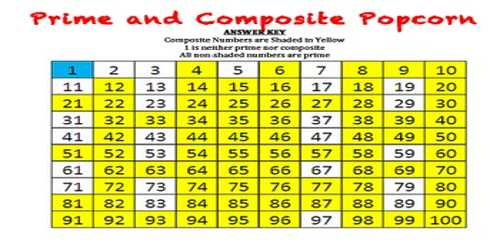Mathematic

# Prime and Composite NumbersPrime and Composite Numbers

A prime number is a whole number that only has two factors which are itself and one. A composite number has factors in addition to one and itself. A Prime Number can be divided evenly only by 1 or itself. And it must be a whole number greater than 1.

The numbers 0 and 1 are neither prime nor composite.

Example:

• 5 can only be divided evenly by 1 or 5, so it is a prime number.
• 6 can be divided evenly by 1, 2, 3 and 6 so it is NOT a prime number, it is a composite number.

All even numbers are divisible by two and so all even numbers greater than two are composite numbers.

All numbers that end in five are divisible by five. Therefore all numbers that end with five and are greater than five are composite numbers.

Prime Numbers

The mathematical definition of a prime number is a number that has exactly two factors (no more or no less). Commonly though people think of prime numbers as being those that can only be divided exactly by 1 and by the number itself.

The prime numbers between 2 and 100 are 2, 3, 5, 7, 11, 13, 17, 19, 23, 29, 31, 37, 41, 43, 47, 53, 59, 61, 67, 71, 73, 79, 83, 89 and 97.Composite Numbers

A natural number that has more than two factors is called a composite number. In other words, a composite number has factors in addition to one and itself.

• All even numbers are divisible by two and so all even numbers greater than two are composite numbers.
• All numbers that end in five are divisible by five. Therefore all numbers that end with five and are greater than five are composite numbers.
• The numbers 0 and 1 are neither prime nor composite.
• If any whole number greater than 1 is not a prime number, then it is a composite number.

Information Source: